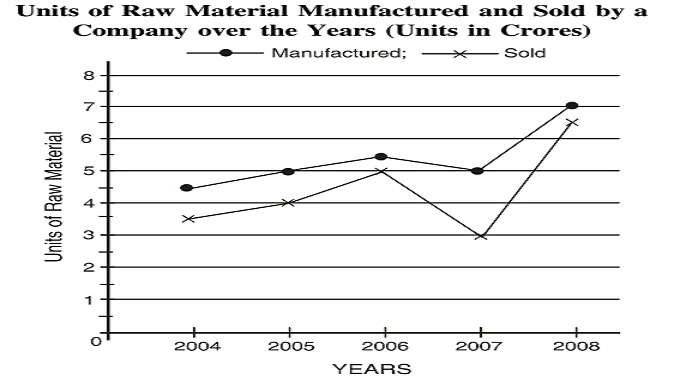## Line chart

#### Data Interpretation

Direction: Study the following Graph carefully and answer the questions given below:1. What is the difference between the number of units manufactured and the number of units sold over the years?

1. Total number of units manufactured = Units manufactured in 2004 + Units manufactured in 2005 + Units manufactured in 2006 + Units manufactured in 2007 + Units manufactured in 2008
Total number of units sold = Units sold in 2004 + Units sold in 2005 + Units sold in 2006 + Units sold in 2007 + Units sold in 2008

##### Correct Option: C

Total number of units manufactured = Units manufactured in 2004 + Units manufactured in 2005 + Units manufactured in 2006 + Units manufactured in 2007 + Units manufactured in 2008
Total number of units manufactured = 4.5 + 5 + 5.5 + 5 + 7 = 27 crores
Total number of units sold = Units sold in 2004 + Units sold in 2005 + Units sold in 2006 + Units sold in 2007 + Units sold in 2008
Total number of units sold = 3.5 + 4 + 5 + 3 + 6.5 = 22 crores
Hence, their difference = 27 crores - 22 crores = 5 crores

1. What is the respective ratio of the number of units manufactured in the year 2004 to the number of units manufactured in the year 2008?

1. Required ratio = number of units manufactured in the year 2004 / number of units manufactured in the year 2008

##### Correct Option: D

Required ratio = number of units manufactured in the year 2004 / number of units manufactured in the year 2008
Required ratio = 4.5 : 7 = 45 : 70 = 9 : 14

1. What is the average number of units sold over the years?

1. Average number of units sold = ( Units sold in 2004 + Units sold in 2005 + Units sold in 2006 + Units sold in 2007 + Units sold in 2008 ) / 5

##### Correct Option: B

Average number of units sold = ( Units sold in 2004 + Units sold in 2005 + Units sold in 2006 + Units sold in 2007 + Units sold in 2008 ) / 5
Average number of units sold = (3.5 + 4 + 5 + 3 + 6.5)/5 = 22/5 = 4.4 crores

1. What is the approximate percent increase in the number of units sold in the year 2008 from the previous year?

1. Required percentage of increase = (number of units sold in the year 2008 - number of units sold in the year 2007) x 100 / number of units sold in the year 2007

##### Correct Option: D

Required percentage of increase = (number of units sold in the year 2008 - number of units sold in the year 2007) x 100 / number of units sold in the year 2007
Required percentage of increase = (6.5 - 3) x 100/3 = 3.5 x 100/3 = 117%

1. What is respective ratio of the difference between the number of units manufactured and sold in the year 2006 to the difference between the number of units manufactured and sold in the year 2007?

1. Required ratio = (number of units manufactured in the year 2006 - number of units sold in the year 2006)/ (number of units manufactured in the year 2007 - number of units sold in the year 2007)

##### Correct Option: B

Required ratio = (number of units manufactured in the year 2006 - number of units sold in the year 2006)/ (number of units manufactured in the year 2007 - number of units sold in the year 2007)
Required ratio = (5.5 - 5) / (5 - 3) = 0.5 / 2 = 1 / 4 = 1 : 4